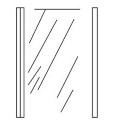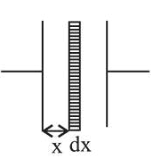# A parallel plate capacitor has plates of area A separated by distance ' d ' between them.

Question:

A parallel plate capacitor has plates of area $A$ separated by distance ' $d$ ' between them. It is filled with a dielectric which has a dielectric constant that varies as $k(x)=K(1+\alpha x)$ where ' $x$ ' is the distance measured from one of the plates. If $(\alpha d)<1$, the total capacitance of the system is best given by the expression:1. (1) $\frac{A K \in_{0}}{d}\left(1+\frac{\alpha d}{2}\right)$

2. (2) $\frac{A \in_{0} K}{d}\left(1+\left(\frac{\alpha d}{2}\right)^{2}\right)$

3. (3) $\frac{A \in_{0} K}{d}\left(1+\frac{\alpha^{2} d^{2}}{2}\right)$

4. (4) $\frac{A K \in_{0}}{d}(1+\alpha d)$

Correct Option: 1

Solution:

(1) Given, $K(x)=K(1+\alpha x)$

Capacitance of element, $C_{e l}=\frac{K \varepsilon_{0} A}{d x}$

$\Rightarrow C_{e l}=\frac{\varepsilon_{0} K(1+\alpha x) A}{d x}$

$\therefore \int d\left(\frac{1}{C}\right)=\frac{1}{C_{e l}}=\int_{0}^{d}\left(\frac{d x}{\varepsilon_{0} K A(1+\alpha x)}\right)$

$\Rightarrow \frac{1}{C}=\frac{1}{\varepsilon_{0} K A \alpha}[\ln (1+\alpha x)]_{0}^{d}$$\Rightarrow \frac{1}{C}=\frac{1}{\varepsilon_{0} K A \alpha} \ln (1+\alpha d)[\alpha \mathrm{d} \ll<1]$

$=\frac{1}{\varepsilon_{0} K A \alpha}\left[\alpha d-\frac{\alpha^{2} d^{2}}{2}\right]$

$=\frac{1}{\varepsilon_{0} K A}\left[1-\frac{\alpha d}{2}\right]$

$\therefore C=\frac{\varepsilon_{0} K A}{d\left(1-\frac{\alpha d}{2}\right)} \Rightarrow C=\frac{\varepsilon_{0} K A}{d}\left(1+\frac{\alpha d}{2}\right)$